Study Guide

# Fluids - Archimedes' Principle

## Archimedes' Principle

### Archimedes and the Crown of Gold

Ever heard the expression Eureka? According to the Merriam-Webster dictionary, we use eureka to "express triumph on a discovery." It is derived from the Greek word heurēka (εὕρηκα), which means "I have found it!"

In the 3rd century BCE, there lived a great inventor, mathematician, and scientist named Archimedes. He was given the task of checking to see whether Goldsmith Crook (Mechanic Crook's ancestor) had mixed in silver while fabricating King Hiero II's gold crown. People knew Archimedes for his genius (he was a sharp fella), and apparently, people knew Goldsmith Crook for his unscrupulous methods. This means that King Hiero must have been awesome at picking up on dishonesty or simply living with an extreme sense of paranoia.

Anyway, gold has a very high density, the density of gold is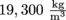. It doesn't matter what shape gold is in, its density will always be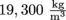.

Archimedes decided that in order to determine whether the King's crown was made of pure gold, he would determine the crown's density.

*Pause*

How do we measure density again? Oh, yes, we remember this. Density equals mass divided by volume, or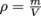. It's easy to determine an object's mass. The problem Archimedes had was figuring out the crown's volume. It's not like it was a cubic, cylinder, or any other regular solid.

*Now back to the story*

Archimedes thought really hard. Melting the crown into a sphere of gold to calculate its volume could work, but would ultimately destroy the crown. King Hiero urged Archimedes to find another way. Goldsmith Crook kept assuring the King the crown was made of pure gold. So what did Archimedes do? Like John Wayne a few centuries later, Archimedes took a bath.

Archimedes noticed the level of water rose while he stepped into his bath. It was precisely at this moment that he had an epiphany. The water level was the key.

The water level had risen by a very specific amount: an amount based on his body's own volume. Logically, then, a crown submerged in water would displace water by an amount equal to the crown's volume. In essence, he could find V.

Archimedes got so excited that he ran down the streets, naked, shouting, "Eureka! Eureka!" Looks like things were dealt with differently back then because he didn't get arrested.

And what happened to Goldsmith Crook? We don't know. We do know that he lived up to his name, Crook, however. The King's crown was not pure gold, but a mixture of gold and silver.

### Buoyancy and Buoyant Force

Archimedes' story illustrates a phenomenon known as buoyancy. Buoyancy is the ability to float.

If we drop a ping-pong ball into a bucket of water, the ball floats. If we drop a golf ball into the bucket, it sinks. What's the difference?

The density of the ping pong ball is less than the density of water, and the density of the golf ball is greater than the density of water, however, both experience a buoyant force. This force was present when Archimedes stepped into his bath before running down the streets naked. An object submerged in a fluid displaces an amount of fluid equal to the object's volume, and the displaced fluid pushes upwards on the object. This is buoyant force. The upward push by the fluid equals the weight of the displaced fluid itself. If the weight of the displaced fluid is greater than the weight of the object, then the object floats. If not, it sinks.

Weight is a force. The weight of displaced water pushing upward is known as Archimedes' Principle, which is illustrated in the following diagram. It's the very definition of the buoyant force.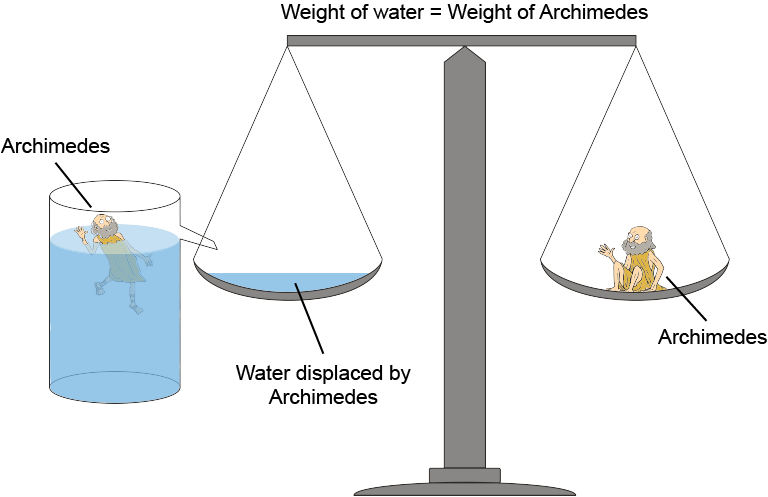Don't forget that it's the weight of the fluid displaced by the object that corresponds to the buoyant force, not the weight of the object.

Objects seem to have different weights in various fluids because of the net difference between the force of gravity pulling down and the buoyant force pushing up . That's why we feel like we weigh less in a pool: the normal force our feet feel at the bottom of the pool is smaller than the normal force they feel on dry land.

The force diagram for this system is the force of gravity pointing downward as usual and the same size as usual (mg), but instead of one normal force upward, there's a buoyant force upward and a smaller normal force, both of which added together balance the force of gravity.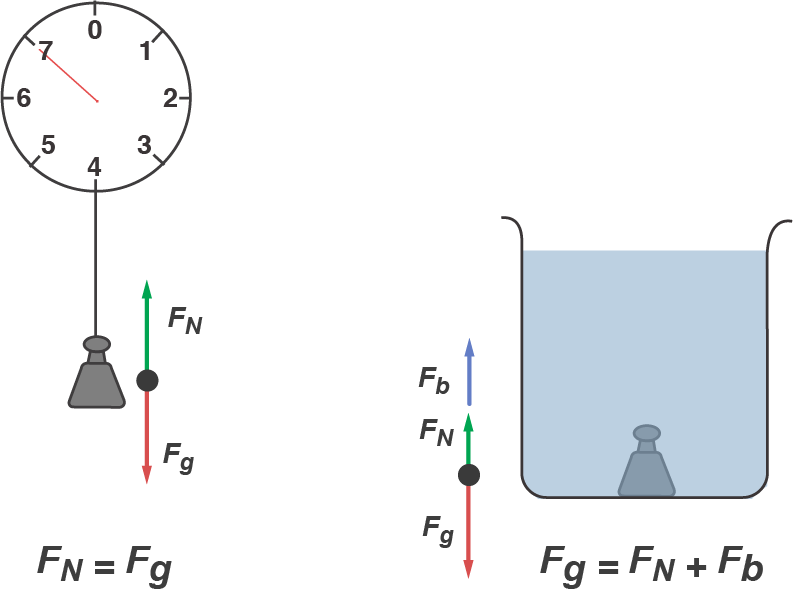To rephrase the very same idea, note that the buoyant force is identical for any two objects of the same size. Density affects the force of gravity of the object itself but not the weight of displaced water. So density also determines whether the object floats or sinks, not the buoyant force.

### Hydrostatic Equilibrium

Must all objects float or sink? Nope. They can just…hang. Literally.

An object in a fluid that doesn't sink all the way down, nor float at the surface, is said to be in equilibrium. If an object is in equilibrium, then the forces upon it are balanced: the force of gravity is equal to (and opposite in direction from) the buoyant force upon it.

Hydrostatic equilibrium refers to the state of an object that doesn't move while submerged in another fluid. In this case, the upward buoyant force Fb of a fluid is equal to its weight Fg while submerged in another fluid. For example, think of oil and water. Oil has a lower density than water, so it floats. The following diagram shows three cases of hydrostatic equilibrium with three balls of different densities.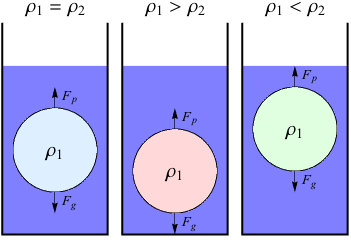Remember that ultimately, an object's buoyancy is determined by its density. This diagram uses ρ to describe density. The density of the object is ρ1, and the density of the fluid is ρ2.

We are all familiar with situations where ρ1 > ρ2, like dropping an iPhone into the toilet. Situations where ρ1 < ρ2 are also common, such as a beach ball floating on water. When ρ1 = ρ2, an object or other fluid has the same density as its surrounding fluid. This is known as neutral buoyancy.

Here's a force diagram for a floating object in equilibrium. Note that the buoyant force (the green arrow) is the same size as the force of gravity (the red arrow), which indicates that the weight of displaced water equals the force of gravity on the object. If the object was held beneath the water and then released, then the buoyant force would be larger than the force of gravity and propel the object up to the surface to find equilibrium.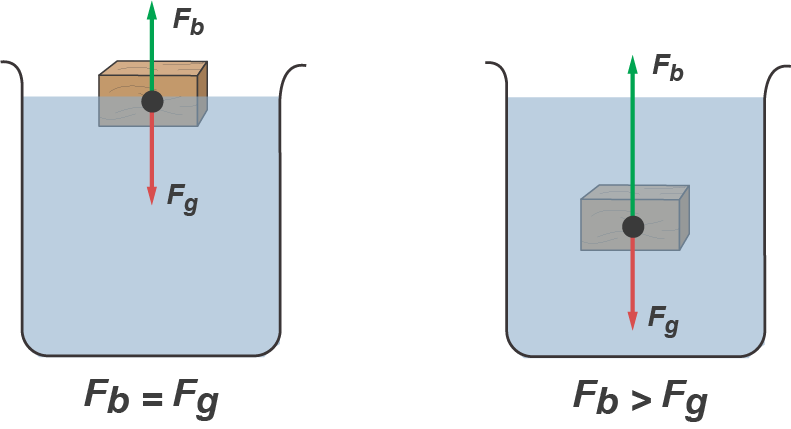We'll learn how to calculate the volume of displaced water for a floating object (using force diagrams and density formulas) later on. Patience, patience.

Oh, never mind. We'll do it now.

A 2.7g ping pong ball floats on a bowl of water. How much water does it displace?

First step, let's gather information to see if we have enough to work with. Since the ping pong ball is not accelerating, we know that Fg = Fb, and we know how to calculate the size of that force with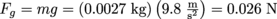. Pretty small.

The buoyant force is also 0.026 N because the two forces are balanced. What now? We know the density of water is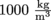. The buoyant force is the weight of the displaced water, or mwg, so the displaced water is also 0.027 g. Now we can use the density of water to find the volume displaced: V =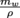= 2.7 × 106 m3, or 2.7 cm3. Ta-da.

If we were given the volume of the ping pong ball as 33.5 cm3 (or the radius with which we could calculate the volume), then we could compare the ratio of out to in and say that the ping pong ball is only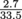submerged, or 8.1%.

### Surface Tension

Anyone who's watched waterbugs on a pond before has an idea of what surface tension is.

These insects are actually called water striders. Since their density is greater than water's, the strider should sink. How do we explain this not-exactly-magical phenomenon?

Imagine a drop of water with all of its 1021 molecules. These molecules attract each other, if they didn't, there would be no drop of water. In physics terms, we'll say these molecules exert forces on each other that "pulls" them together into a spherical shape.

Remembering Newton's Third Law, however, we know that for every force, there exists an equal and opposite force. The drop of water is no exception. Each force exerted by a molecule in a drop of water ends up canceling another force by another molecule in the drop, so these water molecules just float around…la di da…not tied to anything. Except for the molecules at the edges: the surface.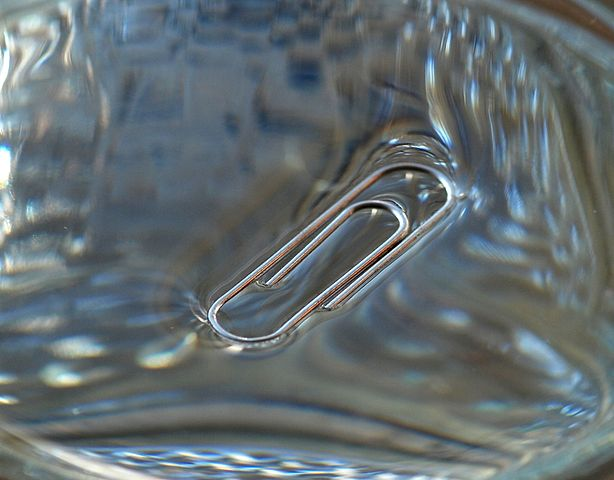(Source)

Those surface molecules have unbalanced forces. Surface molecules have tons of molecules on one side but not the other, the ton of molecules on one side pulls the surface molecules into the drop of water and holds them tight. This creates a kind of tension similar to a membrane. This is how we can "float" a needle or the like on the surface of the water despite the difference in density, the needle just rests on top of this tension.

The water strider does the same thing: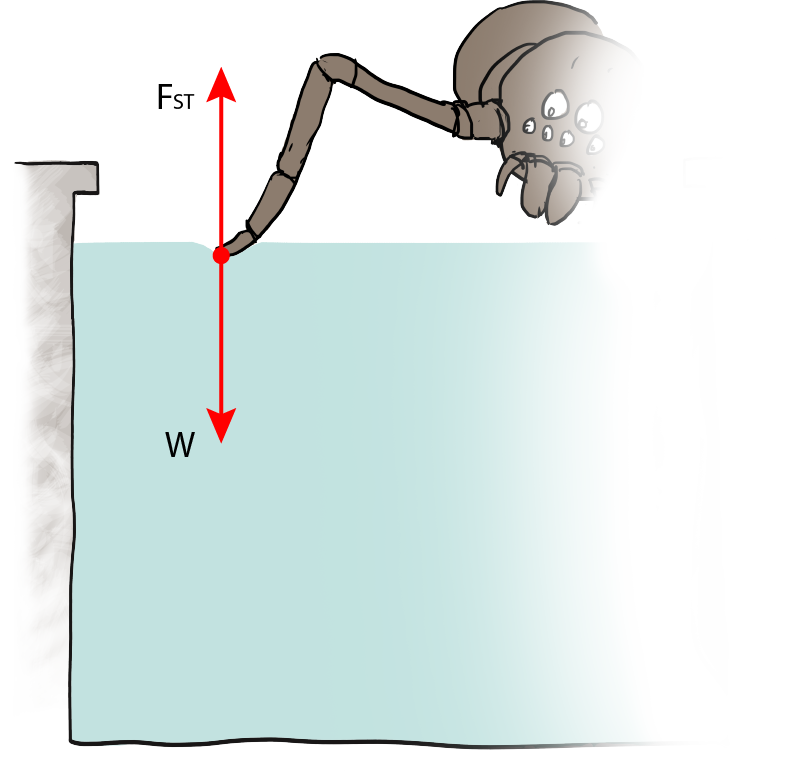The force diagram for each foot of the water strider would include gravity downwards and surface tension upwards. The two balance each other. There's no buoyant force because no water is displaced, no part of the water strider is below the water's surface.
If the insect was larger and had a thicker leg, then FST would have to be larger to compensate for the extra mass added to the weight. That explains the size of water striders, and why we can't stride on water ourselves. Pity.

By definition, surface tension is force divided by a unit of length, so the SI unit is Newtons per meter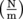. To find the force, we multiply by the length in contact with the surface, the part of the leg touching the water in the case of the strider.

### Common Mistakes

Any time there is a displaced volume of fluid, the buoyant force is present. And any time the object is supported by the surface tension of a fluid (on a surface, or surface area), the force from surface tension is present.

We think of volume as the entire space occupied by a 3D object, and the surface area as the boundary that defines the object's space. Visualize the surface area of a single drop of a fluid by picturing the object wrapped in a thin layer of plastic. If we peel off the plastic and stretch it out, we're left with a 2D surface.

### Brain Snack

Water bugs. Some have cup-shaped feet, which explain their seemingly unnatural buoyancy. Maybe if we had boat-feet we could walk or "stride" on water too. Aren't they cute?